Documentation

# meshQuality

Evaluate shape quality of mesh elements

## Syntax

``Q = meshQuality(mesh)``
``Q = meshQuality(mesh,elemIDs)``
``Q = meshQuality(___,'aspect-ratio')``

## Description

example

````Q = meshQuality(mesh)` returns a row vector of numbers from 0 through 1 representing shape quality of all elements of the mesh. Here, 1 corresponds to the optimal shape of the element.```

example

````Q = meshQuality(mesh,elemIDs)` returns the shape quality of the specified elements.```

example

````Q = meshQuality(___,'aspect-ratio')` determines the shape quality by using the ratio of minimal to maximal dimensions of an element. The quality values are numbers from 0 through 1, where 1 corresponds to the optimal shape of the element. Specify `'aspect-ratio'` after any of the previous syntaxes.```

## Examples

collapse all

Evaluate the shape quality of the elements of a 3-D mesh.

Create a PDE model.

`model = createpde;`

Include and plot the following geometry.

```importGeometry(model,'PlateSquareHoleSolid.stl'); pdegplot(model)```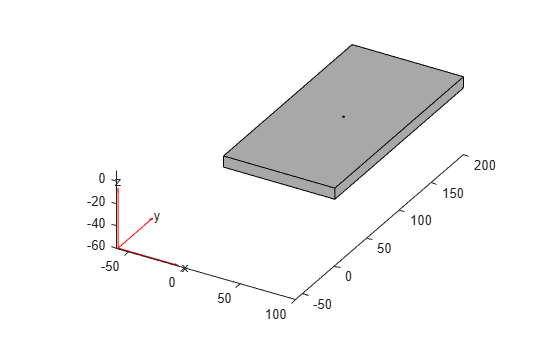Create and plot a coarse mesh.

`mesh = generateMesh(model,'Hmax',35)`
```mesh = FEMesh with properties: Nodes: [3x487 double] Elements: [10x213 double] MaxElementSize: 35 MinElementSize: 17.5000 MeshGradation: 1.5000 GeometricOrder: 'quadratic' ```
`pdemesh(model)`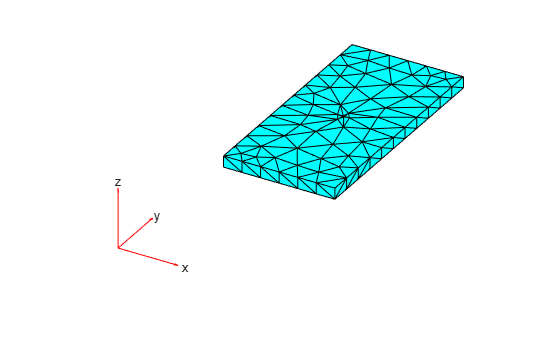Evaluate the shape quality of all mesh elements. Display the first five values.

```Q = meshQuality(mesh); Q(1:5)```
```ans = 1×5 0.3079 0.2917 0.6189 0.6688 0.5571 ```

Find the elements with the quality values less than 0.2.

`elemIDs = find(Q < 0.2);`

Highlight these elements in blue on the mesh plot.

```pdemesh(mesh,'FaceAlpha',0.5) hold on pdemesh(mesh.Nodes,mesh.Elements(:,elemIDs),'FaceColor','blue','EdgeColor','blue')```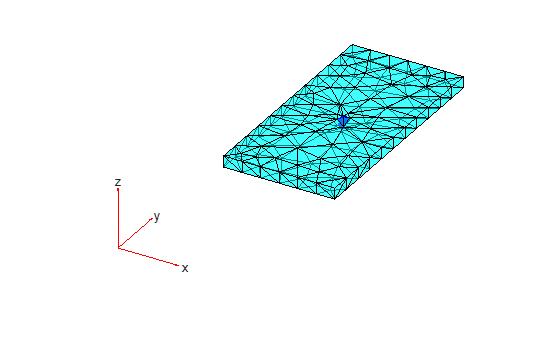Plot the element quality in a histogram.

```figure hist(Q) xlabel('Element Shape Quality','fontweight','b') ylabel('Number of Elements','fontweight','b')```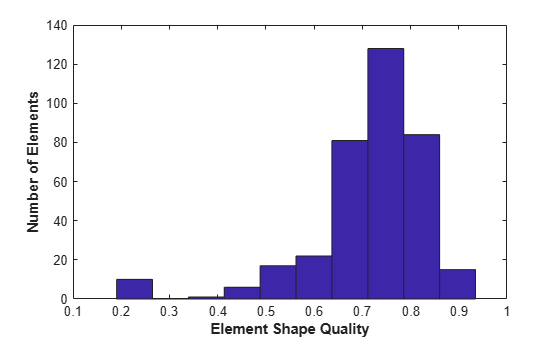Find the worst quality value.

`Qworst = min(Q)`
```Qworst = 0.1691 ```

Find the corresponding element IDs.

`elemIDs = find(Q==Qworst)`
```elemIDs = 1×2 10 136 ```

Evaluate the shape quality of the elements of a 2-D mesh.

Create a PDE model.

`model = createpde;`

Include and plot the following geometry.

```importGeometry(model,'PlateSquareHolePlanar.stl'); pdegplot(model)```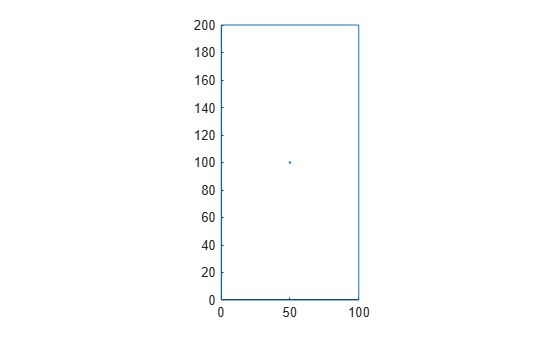Create and plot a coarse mesh.

`mesh = generateMesh(model,'Hmax',20)`
```mesh = FEMesh with properties: Nodes: [2x286 double] Elements: [6x126 double] MaxElementSize: 20 MinElementSize: 10 MeshGradation: 1.5000 GeometricOrder: 'quadratic' ```
`pdemesh(model)`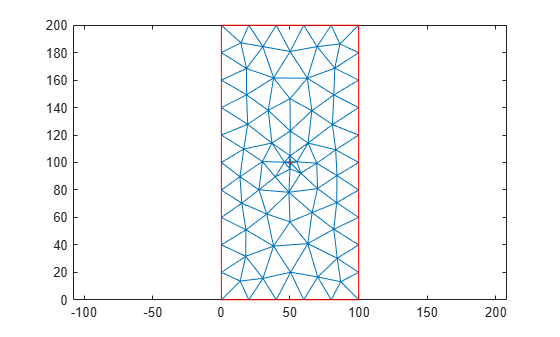Find the IDs of the elements within a box enclosing the center of the plate.

`elemIDs = findElements(mesh,'box',[25,75],[80,120]);`

Evaluate the shape quality of these elements.

`Q = meshQuality(mesh,elemIDs)`
```Q = 1×12 0.2980 0.8253 0.2994 0.6581 0.7838 0.6104 0.3992 0.6921 0.2948 0.5726 0.7016 0.5669 ```

Find the elements with the quality values less than 0.4.

`elemIDs04 = elemIDs(Q < 0.4)`
```elemIDs04 = 1×4 9 19 69 83 ```

Highlight these elements in green on the mesh plot. Zoom in to see the details.

```pdemesh(mesh,'ElementLabels','on') hold on pdemesh(mesh.Nodes,mesh.Elements(:,elemIDs04),'EdgeColor','green') zoom(10)```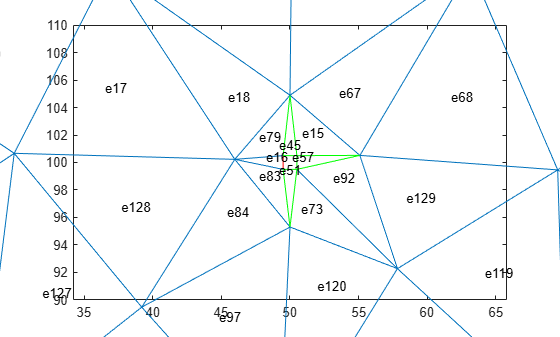Determine the shape quality of mesh elements by using the ratios of minimal to maximal dimensions.

Create a PDE model and include the L-shaped geometry.

```model = createpde(1); geometryFromEdges(model,@lshapeg);```

Generate the default mesh for the geometry.

`mesh = generateMesh(model);`

View the mesh.

`pdeplot(model)`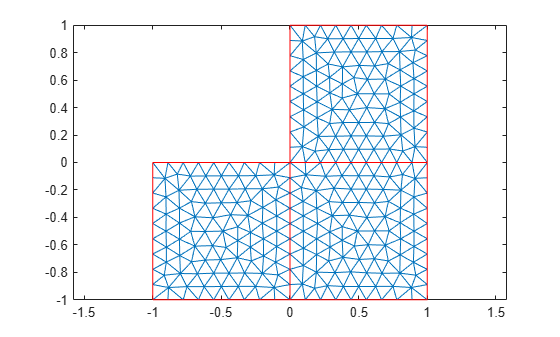Evaluate the shape quality of mesh elements by using the minimal to maximal dimensions ratio. Display the first five values.

```Q = meshQuality(mesh,'aspect-ratio'); Q(1:5)```
```ans = 1×5 0.8339 0.7655 0.7755 0.8301 0.8969 ```

Evaluate the shape quality of mesh elements by using the default setting. Display the first five values.

```Q = meshQuality(mesh); Q(1:5)```
```ans = 1×5 0.9837 0.9605 0.9654 0.9829 0.9913 ```

## Input Arguments

collapse all

Mesh object, specified as the `Mesh` property of a `PDEModel` object or as the output of `generateMesh`.

Example: `model.Mesh`

Element IDs, specified as a positive integer or a matrix of positive integers.

Example: `[10 68 81 97 113 130 136 164]`

## Output Arguments

collapse all

Shape quality of mesh elements, returned as a row vector of numbers from 0 through 1. The value 0 corresponds to a deflated element with zero area or volume. The value 1 corresponds to an element of optimal shape.

Example: [0.9150 0.7787 0.9417 0.2744 0.9843 0.9181]

Data Types: `double`

 Knupp, Patrick M. "Matrix Norms & the Condition Number: A General Framework to Improve Mesh Quality via Node-Movement." In Proceedings, 8th International Meshing Roundtable. Lake Tahoe, CA, October 1999: 13-22.

##### Support평가판 신청